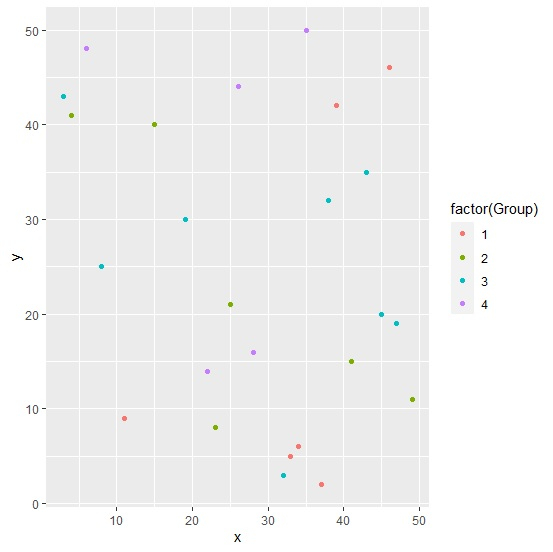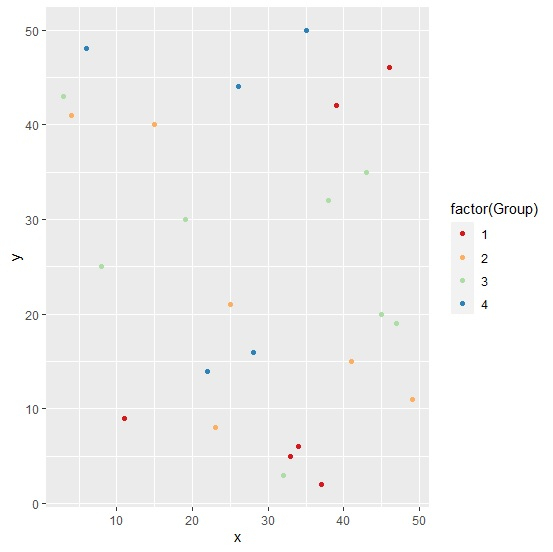# How to change the color of points for ggplot2 scatterplot using color brewer in R?

To change the color of points for ggplot2 scatterplot using color brewer in R, we can follow the below steps −

• First of all, create a data frame.
• Then, create the point chart with default colors.
• After that, use scale_colour_brewer function to create the point chart.

## Create the data frame

Let's create a data frame as shown below −

Live Demo

x<-sample(1:50,25)
y<-sample(1:50,25)
Group=sample(1:4,25,replace=TRUE)
df<-data.frame(x,y,Group)
df

On executing, the above script generates the below output(this output will vary on your system due to randomization) −

   x  y  Group
1  23  8  2
2  49  11 2
3  19  30 3
4  35  50 4
5  41  15 2
6  11  9  1
7  3   43 3
8  34  6  1
9  33  5  1
10 32  3  3
11 37  2  1
12 4   41 2
13 6   48 4
14 26  44 4
15 15  40 2
16 47  19 3
17 28  16 4
18 43  35 3
19 39  42 1
20 38  32 3
21 8   25 3
22 22  14 4
23 46  46 1
24 25  21 2
25 45  20 3

## Create the point chart with default colors

Loading ggplot2 package and creating the point chart between x and y based on values in Group column −

x<-sample(1:50,25)
y<-sample(1:50,25)
Group=sample(1:4,25,replace=TRUE)
df<-data.frame(x,y,Group)
library(ggplot2)
ggplot(df,aes(x,y,col=Group))+geom_point()

#### Output## Create the point chart with color brewer

Use scale_colour_brewer function of the ggplot2 package to create the point chart between x and y based on values in Group column −

x<-sample(1:50,25)
y<-sample(1:50,25)
Group=sample(1:4,25,replace=TRUE)
df<-data.frame(x,y,Group)
library(ggplot2)
ggplot(df,aes(x,y,col=factor(Group)))+geom_point()+scale_colour_brewer(palette="Spec
tral")

#### Output